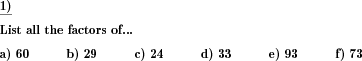Custom math worksheets at your fingertips# Details for problem "Factors of a number"

Quickname: 1435

Elementary School, Primary School, Junior High School, Middle School, High School.

## Summary

For a given number, all factors have to be listed.

## Example## Description

The factor is an important topic of mathematics to learn. The factors of a given number are all values that divide the given number without a remainder.

These problem can be used for different grades to practice the skill of finding factors of given numbers in groups, or independent activity. Before starting algebraic factoring, it’s a good strategy to recall these factors.

By using this problem, the following objectives will be achieved.

• Multiplication and division concepts will be revised in detail.
• Practice and understanding of divisibility rules.
• Strong concepts of factoring given positive numbers will be achieved through practice.
• The practice of these worksheets will also lay the foundation for factoring integers.
• Provide a basis to achieve a perfect level of understanding the math topics like Prime Factor, Greatest Common Factors.
• Factors proficiency is a prerequisite for many algebraic operations such as Algebraic Expressions Factors.

In each question, a whole positive number are given. All whole positive factors for this number have to be determined.

The number of problems and the number range for the number to be worked on can be chosen. Each worksheet can be generated with problems starting from 1 problem and a maximum of 10 problems per worksheet. The number range is up to 100 starting from 10 and up to 100.

The answer key will list the factorization of each given number in the question.

Download free printable worksheets for this math problem here. The worksheet contains the problems only, the solution sheet includes the answers. Just click on the respective link.

•Worksheet 1Solution sheet with answers
•Worksheet 2Solution sheet with answers
•Worksheet 3Solution sheet with answers

If you can not see the solution sheets for download, they may be filtered out by an ad blocker that you may have installed. If this is the case, please allow ads for this page and reload the page. The solution sheets will then reappear.

• Do these sample worksheets do not really fit?
• Do you need more math worksheets, with a different level of difficulty?
• Would you like to combine different problems on a worksheet and adjust them to your needs?
• As a teacher, you can put together your own worksheets using the automatically generated math problems provided.
With a free initial credit, you can start creating your own math worksheets in a few minutes.

You can try it for free! Register here, to create custom worksheets now!

## Customization options for this problem

Parameter
Possible values
Number of problems
1, 2, 3, 4, 5, 6, 7, 8, 9, 10
Number range
10, 20, 30, 40, 50, 100

## Similar problems

Remark
Description
Find gcd and lcm of two numbers
For two given numbers, the gcd and lcm are determined by comparing lists of multiples or divisors